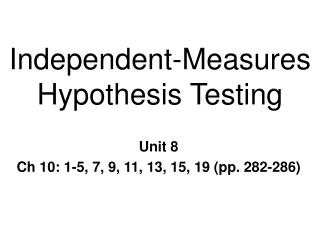# Independent-Measures Hypothesis Testing - PowerPoint PPT PresentationDownload PresentationIndependent-Measures Hypothesis Testing

Independent-Measures Hypothesis Testing
Download Presentation## Independent-Measures Hypothesis Testing

- - - - - - - - - - - - - - - - - - - - - - - - - - - E N D - - - - - - - - - - - - - - - - - - - - - - - - - - -
##### Presentation Transcript

1. Independent-Measures Hypothesis Testing Unit 8 Ch 10: 1-5, 7, 9, 11, 13, 15, 19 (pp. 282-286)

2. Comparing 2 sets of data • 2 general research strategies • data sets come from 2 separate groups • independent samples • between groups design • 2 sets of data from 1 group • dependent or related samples • matched-subjects (2 related groups) • within subjects design ~

3. Independent Measures Hypothesis Test • Select 2 independent samples • are they from same population? • Experiment • select 2 samples • 1 receives treatment • are the samples the same? ~

4. Experimental Outcomes • Do not expect to be exactly equal • sampling error • How big a difference to reject H0 ? ~

5. Hypotheses: Independent Measures • Nondirectional • H0: m1 - m2 = 0 H1: m1 - m2 0 • or H0: m1 = m2 H1: m1m2 • Directional (depends on prediction ) • H0: m1 - m2 < 0 H1: m1 - m2 > 0 • or H0: m1<m2 H1: m1 > m2 • no value specified for either • Group 1 scores = Group 2 scores~~

6. Sample statistic: t test: Independent Samples • Same basic structure as single sample • Independent samples [df = n1 + n2 - 2]

7. The Test Statistic • Since m1 - m2 = 0 [df = n1 + n2 - 2]

8. Estimated Standard Error • *Standard error of difference between 2 sample means • must calculate s2p first ~

9. Pooled Variance (s2p) • Average of 2 sample variances • weighted average if n1n2 • if n1 =n2

10. The Test Statistic: Assumptions 1. Samples are independent 2. Samples come from normal populations 3. Assume equal variance s21 = s22 • does not require s21 = s22 • homogeneity of variance • t test is robust • violation of assumptions • Little effect on P(rejecting H0) ~

11. Example: Independent Samples • Is exam performance affected by how much sleep you get the night before a test? • Dependent variable? • independent variable? • Grp 1: 4 hrs sleep (n = 6) • Grp 2: 8 hrs sleep (n = 6) ~

12. Example: n1 = n2 1. State Hypotheses H0: m1 - m2 = 0 or H0: m1= m2 H1: m1 - m2 ¹ 0 or H1: m1 ¹m2 2. Set criterion for rejecting H0: nondirectional a = .05 df = (n1 + n2 - 2) = (6 + 6 - 2) = 10 tCV.05 =

13. Example: n1 = n2 3. select sample, compute statistics do experiment • mean exam scores for each group • Group 1: M1 = 15 ; s1 = 4 • Group 2: M2 = 19; s2 = 3 • compute • s2p • s M1-M2 • tobs~

14. Example: n1 = n2 • compute s2p

15. Example: n1 = n2 • compute

16. Example: n1 = n2 • compute test statistic

17. Example: n1 = n2 4. Decision? • Is tobs in critical region? • No, fail to reject H0 • If directional test or change level of significance • change critical value of t (tcv) • just like other tests ~

18. Pooled Variance: n1¹n2 • Unequal sample sizes • weight each variance • bigger n ---> more weight

19. Example: n1¹n2 Supplementary Material • What effect does the amount of sleep the night before an exam have on exam performance? • Dependent variable • independent variable • Grp 1: 4 hrs sleep (n = 6) • Grp 1: 8 hrs sleep (n = 7) ~

20. Example: n1¹n2 1. State Hypotheses H0:m1 = m2 or m1 - m2 = 0 H1:m1¹ m2 or m1 - m2 ¹ 0 2. Set criterion for rejecting H0: nondirectional a = .05 df = (n1 + n2 - 2) = (6 + 7 - 2) = 11 tCV = + 2.201 ~

21. Example: n1¹n2 3. select sample, compute statistics do experiment mean exam scores for each group • Group 1: M1 = 14 ; s1= 3 • Group 2: M2 = 19; s2= 2 • compute • s2pooled • sM1- M2 • tobs~

22. Example: n1¹n2 • compute s2pooled • compute • compute test statistic [df = n1 + n2 - 2]

23. Example: n1¹n2 4. Interpret Is tobsbeyond tCV? If yes, Reject H0.